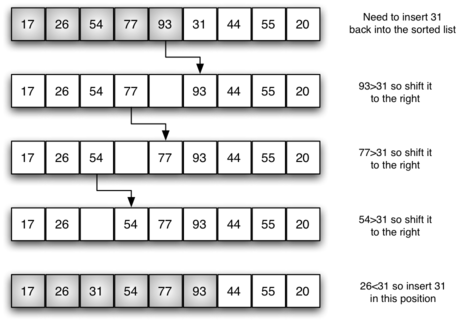# Data Structure : Binary Search Tree## Binary search trees (BSTs) are very easy to understand. Here we start with a root node n with value x, where the left subtree to n contains nodes with values < x and the right subtree contains nodes whose value are >=x. Here we are learn about Insertion into BST, Searching, Deletion from the tree and more about Binary Search Tree.

BSTs are of interest because they have operations which are favourably fast: insertion, look up, and deletion can be in O(log n) time. It is important to note that the O(log n) times for these operations can only be attained if the BST is reasonably balanced, for a tree data structure with self balancing properties see AVL tree.

Binary Search Tree, is a node-based binary tree data structure which has the following properties:

• The left subtree of a node contains only nodes is less than its parent node.
• The right subtree of a node contains only nodes is greater than or equals to its parent node.
• The left and right subtree each must also be a binary search tree.
There must be no duplicate nodes.

In the following examples you can assume, unless used as a parameter alias### The Complexity:

In Binary Search is applicable on Sort Array (Usual we can say a BST). In this algorith a array divide into two sub-array repeat this step until the array contains single element

Suppose A[] of length N

then,

N/2k =1 (It means how many times we divide N by 2 to get 1)

N = 2k

Taking log2 on both side

log N = log 2k

log N = k log22

log N = k (since log22 = 1)

Hance

k = log N

Finally, The complexity of Binary Search in O(log n)

## Insertion

As discussed above insertion is an O(log n) provided that the tree is moderately balanced.

``````1- algorithm Insert(value)
2- Pre: value has passed custom type checks for type T
3- Post: value has been placed in the correct location in the tree
4- if root = ?
5- root ? node(value)
6- else
7- InsertNode(root, value)
8- end if
9- end Insert``````
``````1- algorithm InsertNode(current, value)
2- Pre: current is the node to start from
3- Post: value has been placed in the correct location in the tree
4- if value < current.Value
5- if current.Left = ?
6- current.Left ? node(value)
7- else
8- InsertNode(current.Left, value)
9- end if
10- else
11- if current.Right = ?
12- current.Right ? node(value)
13- else
14- InsertNode(current.Right, value)
15- end if
16 -end if
17- end InsertNode``````##### Vijay Pal
Founder Of STUDYZONE24.COM | Learning is a journey, let’s learn together.

Related articles
•## Data Structure : Sorting Algorithms

•## Microservices Architecture Style

•## Sum Of Two Elements In Array Equal To k

•## Find All Pair of Elements whose Sum is Equal to K

•## Merge Sort Algorithm | Complexity of Merge Sort Algorithm

•## Bubble Sort Algorithm | Complexity of Bubble Sort Algorithm

•